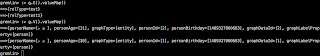#### How Can I make a statistics,i.e:how many vertexes or edges?

spirit...@...

My graph has about 100 million vertexes and 200 million edges. But if use the following code, it is too slow.
`GraphTraversal<Vertex, Long> countV = traversal.V().count();`
`while (countV.hasNext()){    System.out.println("countV:" + countV.next());}GraphTraversal<Edge, Long> countE = traversal.E().count();while (countE.hasNext()){    System.out.println("countE:" + countE.next());}`

I want to computer the count of vertex or edge directly through Hbase. The following code is:
` SnapshotCounter.HBaseGetter entryGetter = new SnapshotCounter.HBaseGetter();        EntryList entryList = StaticArrayEntryList.ofBytes(                result.getMap().get(Bytes.toBytes("e")).entrySet(),                entryGetter);        StandardTitanTx tx = (StandardTitanTx) graph.newTransaction();        System.out.println("Entry list size: " + entryList.size());        int cnt = 0;//        IDInspector inspector = graph.getIDInspector();        for (Entry entry : entryList) {            RelationCache relation = graph.getEdgeSerializer().readRelation(entry, false, tx);//            Direction direction = graph.getEdgeSerializer().parseDirection(entry);//            System.out.println("Direction is:" + direction.name());//            System.out.println("relation is:" + relation);//            System.out.println("numProperties: " + relation.numProperties());//            Iterator<LongObjectCursor<Object>> longObjectCursorIterator = relation.propertyIterator();//            LongObjectCursor<Object> next = longObjectCursorIterator.next();//            System.out.println("key is:" + next.key);//            System.out.println("value is:" + next.value);//            System.out.println("next.toString is:" + next.toString());            RelationType type = tx.getExistingRelationType(relation.typeId);            Iterator<Edge> edgeIterator1 = type.edges(Direction.BOTH);            while (edgeIterator1.hasNext()){                Edge next11 = edgeIterator1.next();                System.out.println("relType is :" + next11.property("relType"));            }//             if (type.isEdgeLabel() && !tx.getIdInspector().isEdgeLabelId(relation.relationId)){//            if (type.isEdgeLabel() &&  !graph.getIDManager().isEdgeLabelId(relation.relationId) &&//                    !tx.getIdInspector().isRelationTypeId(type.longId())) {            if (type.isEdgeLabel() ) {                cnt++;                System.out.print("isSystemRelationTypeId: ");                System.out.println(graph.getIDManager().isSystemRelationTypeId(relation.typeId));                System.out.print("isEdgeLabelId: ");                System.out.println(graph.getIDManager().isEdgeLabelId(relation.typeId));                System.out.print("type isEdgeLabel: ");                System.out.println(type.isEdgeLabel());                System.out.print("relationId isSystemRelationTypeId: ");                System.out.println(graph.getIDManager().isSystemRelationTypeId(relation.relationId));                System.out.println(entry.getValue().toString());            }        }        System.out.println("Edge count: " + cnt);`

I made a test by making a small graph-- two vertexes and two edges.But I just get the count of the edge is one, expecting two. Is there any problem? Please help....This problem bugs me. Thanks~~~

Join janusgraph-users@lists.lfaidata.foundation to automatically receive all group messages.